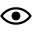## No Title

Author: 965dcca30a18 August 2021

Views: 12

// Implenting Merge sort in C

#include <stdio.h>

// Merge two subarrays L and M into arr

void merge(int arr[], int p, int q, int r) {

// Create L ← A[p..q] and M ← A[q+1..r]

int n1 = q - p + 1;

int n2 = r - q;

int L[n1], M[n2];

for (int i = 0; i < n1; i++)

L[i] = arr[p + i];

for (int j = 0; j < n2; j++)

M[j] = arr[q + 1 + j];

// Maintain current index of sub-arrays and main array

int i, j, k;

i = 0;

j = 0;

k = p;

// Until we reach either end of either L or M, pick larger among

// elements L and M and place them in the correct position at A[p..r]

while (i < n1 && j < n2) {

if (L[i] <= M[j]) {

arr[k] = L[i];

i++;

} else {

arr[k] = M[j];

j++;

}

k++;

}

// When we run out of elements in either L or M,

while (i < n1) { // pick up the remaining elements and put in A[p..r]

arr[k] = L[i];

i++;

k++;

}

while (j < n2) {

arr[k] = M[j];

j++;

k++;

}

}

// Divide the array into two subarrays, sort them and merge them

void mergeSort(int arr[], int l, int r) {

if (l < r) {

// m is the point where the array is divided into Two Subarrays

int m = l + (r - l) / 2;

mergeSort(arr, l, m);

mergeSort(arr, m + 1, r);

merge(arr, l, m, r); // Merge the sorted subarrays

}

}

// Print the array

void printArray(int arr[], int size) {

for (int i = 0; i < size; i++)

printf("%d ", arr[i]);

printf("\n");

}

// Driver program

int main() {

int arr[] = {8, 13, 24, 33, 48};

int size = sizeof(arr) / sizeof(arr);

mergeSort(arr, 0, size - 1);

printf("Sorted array: \n");

printArray(arr, size);

}